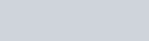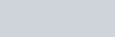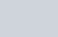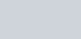Integration can be thought of as the inverse of differentiation.

In the initial video I introduce you to this concept and explain the notation used and run through how we apply the following formulae.

Formulae to remember:

•••What is integration? - Introduction (tutorial 1) | ExamSolutions - youtube Video

Fractional and root type terms

Integrals that contain one term where x is in the denominator can often be rearranged and then integrated. In the video that follows I show you how to do the following.

Examples in the video

1.2.Calculus : Integration : fractional & root types (tutorial 2) : ExamSolutions - youtube Video